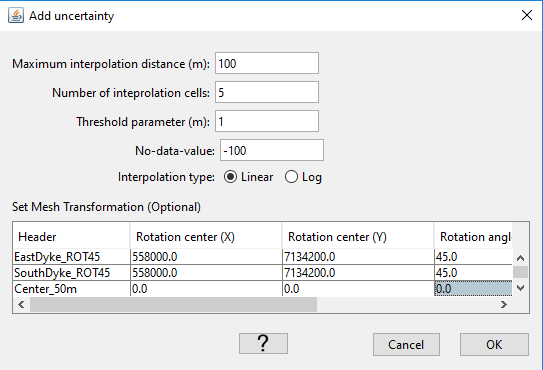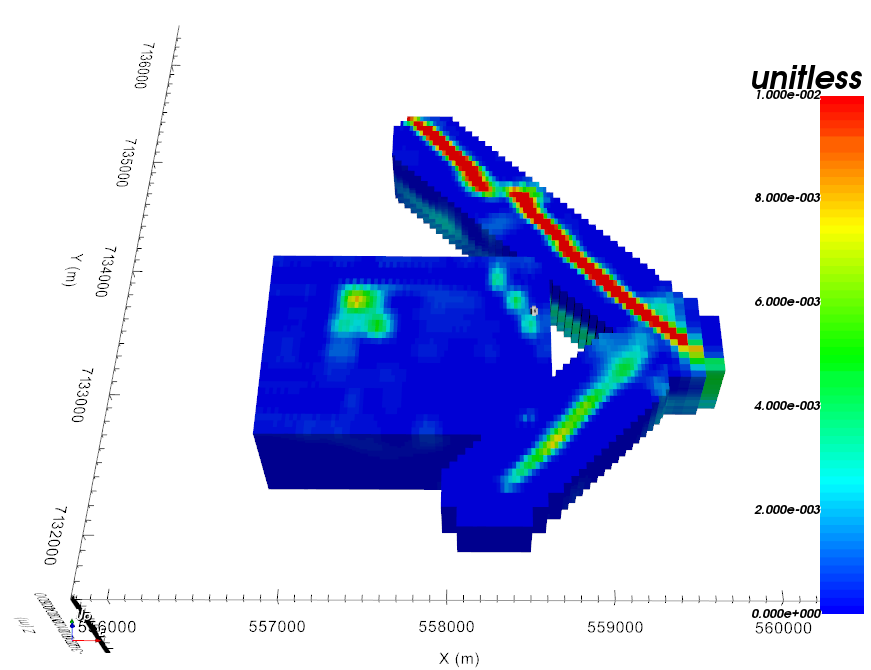# 4.2.9. Merge (Interpolate) 3D models¶

This tool allows the user to merge multiple 3D models onto a given mesh. The operation can be done across meshes with different discretization. The main menu allows the user to specify simple grid transformations as well as interpolation parameters:The following fields control the inverse distance interpolation:

$\mathbf{\bar m} = \frac{\sum_{i=1}^{N} \mathbf{m_i}{r_i}}{\sum_{i=1}^{N} {r_i}}$
${r_i} = \left[ (x(\bar m) - x(m_i))^{2} + (y(\bar m) - y(m_i))^{2} + \delta \right]^{1/2}$

where x and y define the cell center location of model column m in the underlying mesh.

 Options Description Maximum Interpolation Distance Neighboring cells with active property values Interpolation Points Number of neighbors included in the weighted average Threshold parameters Smoothing factor No-data-value Fill values for air cells and empty model Rotation center [X,Y] Easting, Northing position of rotation center Rotation angle Rotation counter-clockwise from East Translation [X,Y] Grid translation distances

Example